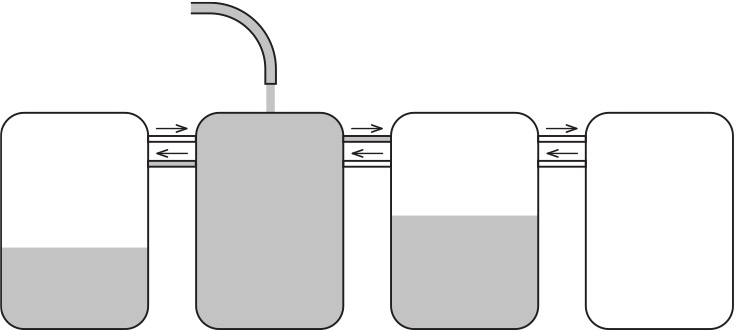시간 제한 메모리 제한 제출 정답 맞은 사람 정답 비율
1 초 512 MB 1 1 1 100.000%

## 문제

It's a little-known fact that Innopolis has a secret factory that produces secret liquid. There are $n$ barrels locating in a row, indexed from 1 to $n$. Barrel $i$ can contain $a_i$ nanoliters of secret liquid.

Barrels are connected with directed pipes, secret liquid can flow only in one direction. For all $i$ from 1 to $n - 1$ there is a pipe from barrel $i$ to barrel $i + 1$ and a pipe from barrel $i + 1$ to barrel $i$. This means there are two pipes between any two consecutive barrels: one for each direction. For each pipe its capacity is known: number of nanoliters of secret liquid that can pass through pipe. When that capacity is reached, liquid can no longer pass through the pipe.

Head of production department wants to choose a single barrel and put a pouring tap above this barrel. When tap is opened, this barrel is filled with secret liquid. After it's fully filled, liquid is going to pass to neighboring barrels through pipes, until either neighboring barrel is filled, or pipe capacity is reached. If pipe capacity wasn't reached, liquid can pass to next barrels, and so on. Process is finished when either all barrels are filled, or pipe capacities are reached, so that no liquid can pass further.Help head of department to choose a starting barrel so that the total volume of secret liquid in all the barrels is as large as possible in the end.

## 입력

First line contains integer $n$ --- number of barrels ($1 \le n \le 5 \cdot 10^5$).

Each of the next $n$ lines contains three integers $l_i$, $r_i$ and $a_i$ --- capacities of pipes connecting barrel $i$ to barrel $i-1$ and $i+1$, respectively, and capacity of barrel $i$ ($0 \le l_i, r_i, a_i \le 10^9$; $l_1 = r_n = 0$).

## 출력

Output a single integer --- total volume of secret liquid in all barrels.

## 서브태스크

번호 배점 제한
1 17

$n \le 100$

2 29

$n \le 1000$

3 31

$n \le 100\,000$

4 23

$n \le 500\,000$

## 예제 입력 1

3
0 2 3
4 0 4
1 0 5


## 예제 출력 1

7


## 예제 입력 2

3
0 10 5
0 10 2
0 0 1


## 예제 출력 2

8


## 힌트

In the first sample it is optimal to place the tap above the second barrel. After the second barrel is filled, liquid pours in the first barrel and fills it fully. The third barrel will remain empty, because the capacity of the pipe from barrel 2 to barrel 3 is zero.

In the second sample you should place the tap above the first barrel. After it's filled, liquid to the second barrel and to the third barrel, filling both of them.

## 채점 및 기타 정보

• 예제는 채점하지 않는다.